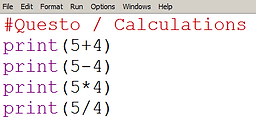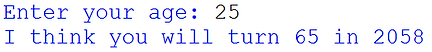top of page

## 1. Creating Calculations

To add in Python use +

To subtract in Python use -

To multiply in Python use *

To divide in Python use /

Task 1 - Create a new Python program and save the file as 4-Calculations.py

Print 4 different calculations - use each operator once.(add/subtract/multiply/divide)

Python is very clever, so don't be afraid to make your own calculations using larger numbers and more than one operator.## 2. Using Inputs and Calculations

You can ask the user to enter numbers by writing input lines.

Python can then perform calculations using the numbers that the user has entered.

Don't forget to add int( and double close brackets when using numbers!

Task 2 - Copy the new code from the picture. Change the text and num1 so you are dividing by 5 not 3.I have cropped the images to make the new code clearer.

## 3. Using Calculations in a Sentence

When we have printed the calculations so far, they have not been very informative!

You can print calculations together with sentences so that they mean more.

Use a comma ( , ) between calculations and sentences.## Challenge Programs

1. Create a new Python program. Save it as '4-Multiplication.py'

2. Add a comment at the top with your name and the date.

3. Write an input line (don't forget int!) that asks the user to enter number 1.

4. Write an input line (don't forget int!) that asks the user to enter number 2.

5. Multiply number 1 and number 2 together and print the answer.

• BONUS: Try to show number 1 and number 2 in the print statement (see practice task 3 to help you).

• Remember: Break up variables in a print line by using commas.

When you run it, it could look something like this:

1. Create a new Python program. Save is as '4-Retirement.py'

2. Add a comment at the top with your name and the date.

3. Write an input line (don't forget int!) that asks the user to enter their age.

4. Print the year that they will turn 65.

(This is slightly tricky. Hint: You need to know their age, and you need to know the current year. You need to subtract something, and you need to add something. Try using scrap paper to help you figure it out.)

• BONUS: Use only one print line.

• BONUS: Try to use only two lines in total.

Remember: Break up variables in a print line by using commas.

When you run it, it could look something like this:bottom of page